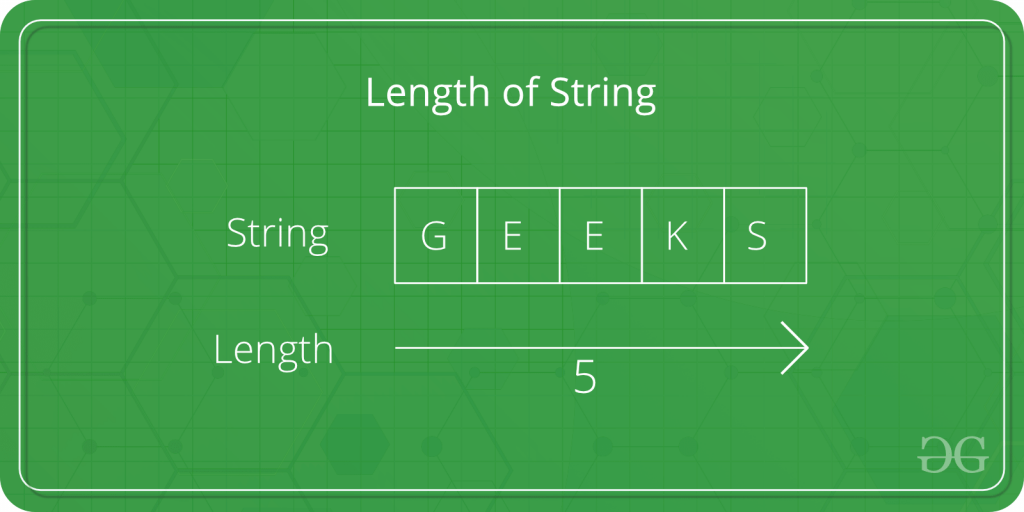Open In App
Related Articles

# C program to find the length of a string

Given a string str. The task is to find the length of the string.Examples

```Input: str = "Geeks"
Output: Length of Str is : 5
Input: str = "GeeksforGeeks"
Output: Length of Str is : 13```

In the below program, to find the length of the string str, first the string is taken as input from the user using scanf in, and then the length of Str is calculated usingand usingmethod .
Below is the C program to find the length of the string.

Example 1: Using loop to calculate the length of string.

## C

 `// C program to find the length of string``#include ``#include ` `int` `main()``{``    ``char` `Str;``    ``int` `i;` `    ``printf``(``"Enter the String: "``);``    ``scanf``(``"%s"``, Str);` `    ``for` `(i = 0; Str[i] != ``'\0'``; ++i);` `    ``printf``(``"Length of Str is %d"``, i);` `    ``return` `0;``}`

Output

`Enter the String: Length of Str is 0`

Time Complexity: O(N)
Auxiliary Space: O(1)

Example 2: Using strlen() to find the length of the string.

## C

 `// C program to find the length of``// string using strlen function``#include ``#include ` `int` `main()``{``    ``char` `Str;``    ``int` `i;` `    ``printf``(``"Enter the String: "``);``    ``scanf``(``"%s"``, Str);` `    ``printf``(``"Length of Str is %ld"``, ``strlen``(Str));` `    ``return` `0;``}`

Output

`Enter the String: Length of Str is 0`

Time Complexity: O(1)
Auxiliary Space: O(1)

Example 3: Using sizeof() to find the length of the string.

## C

 `// C program to find the length of``// string using sizeof() function``#include ``#include ` `int` `main()``{` `    ``printf``(``"Enter the String: "``);` `    ``char` `ss[] = ``"geeks"``;` `    ``printf``(``"%s\n"``, ss);` `    ``// print size after removing null char.``    ``printf``(``"Length of Str is %ld"``, ``sizeof``(ss) - 1);` `    ``return` `0;``}``// This code is contributed by ksam24000`

Output

```Enter the String: geeks
Length of Str is 5```

Time Complexity: O(1)
Auxiliary Space: O(1)

Example 4: Using Pointer Subtraction

## C

 `// C program to find the size of string by pointer``// substraction``#include ` `int` `main()``{``    ``char` `str[] = ``"Geeks"``;``    ``char``* ptr = str;` `    ``// traversing till last pointer``    ``while` `(*ptr) {``        ``ptr++;``    ``}` `    ``printf``(``"Address of start position of string : %x\n"``,``           ``str);``    ``printf``(``"Address of end position of string : %x\n"``, ptr);` `    ``// difference between the address will give the length``    ``// of string` `    ``int` `length = ptr - str;``    ``printf``(``"Length of the string: %d\n"``, length);``    ``return` `0;``}``// contributed by puligokulakishorereddy`

Output

```Address of start position of string : e996300
Address of end position of string : e996305
Length of the string: 5
```

Time Complexity: O(n)
Space Complexity: O(1)

Explanation

1. In the main() function, a character array str[] is defined and initialized with the string “Greeks“.
2. A pointer ptr is declared and initialized with the starting address of the str = e996300 array.
3. The code enters a while loop that iterates until the value pointed to by ptr is not null. In C, the null character ‘\0‘ signifies the end of a string.
4. Inside the loop, the ptr is incremented to point to the next character in the string.
5. After the loop, the code calculates the length of the string by subtracting the starting address of str = e996300 from the final value of ptr = e996305. This pointer subtraction gives the number of elements (characters) between the two addresses

Note: The address may be different in different execution of the above program.